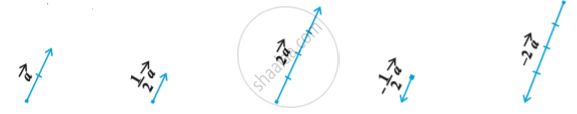# Multiplication of a Vector by a Scalar

#### notes

Let vec a be a given vector and lambda a scalar. Then the product of the vector vec a by the scalar lambda , denoted as lambda vec a , is called the  multiplication of vector vec a by the scalar lambda. lambda vec a is also a vector, collinear to the vector vec a .  The vector lambda vec a  has the direction same (or opposite) to that of vector vec a according as the value of λ is positive (or negative). Also, the magnitude of vector λvec a is |λ| times the magnitude of the vector vec a , i.e.,
|λ vec a| = |λ| |vec a|
A geometric visualisation of multiplication of a vector by a scalar is given in fig.When λ = –1, then λvec a = – vec a, which is a vector having magnitude equal to the magnitude of  and direction opposite to that of the direction of vec a . The vector –vec a is called the negative (or additive inverse) of vector vec a and we always have
vec a + (-vec a) = (-vec a) + vec a = 0
Also, if  λ = 1/|vec a|  ,  provided vec a ≠ 0 i.e.  is not a null vector, then
|λ veca| =|λ| |vec a| = 1/|vec a||vec a| = 1
So, λ vec a represents the unit vector in the direction of vec a. We write it as
hat a = 1/|vec a| vec a

If you would like to contribute notes or other learning material, please submit them using the button below.

### Shaalaa.com

multiplication by Scalar and Position Vector [00:20:59]
S
0%